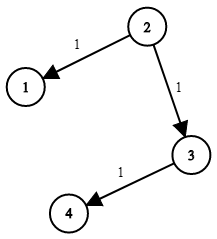# LeetCode解题分享：743. Network Delay Time

#### Problem

There are N network nodes, labelled 1 to N.

Given times, a list of travel times as directed edges times[i] = (u, v, w), where u is the source node, v is the target node, and w is the time it takes for a signal to travel from source to target.

Now, we send a signal from a certain node K. How long will it take for all nodes to receive the signal? If it is impossible, return -1.

Example 1:Input: times = [[2,1,1],[2,3,1],[3,4,1]], N = 4, K = 2
Output: 2

Note:

1. N will be in the range [1, 100].
2. K will be in the range [1, N].
3. The length of times will be in the range [1, 6000].
4. All edges times[i] = (u, v, w) will have 1 <= u, v <= N and 0 <= w <= 100.
##### 解题思路

经典的Dijkstra算法例题。

代码如下：

class Solution {
public:
int networkDelayTime(vector<vector<int>>& times, int N, int K) {
const int inf = INT_MAX;

vector<vector<int>> graph(N, vector<int>(N, inf));

vector<bool> visited(N, false);

vector<int> dist(N, inf);

dist[K - 1] = 0;

for (auto& edge : times)
{
graph[edge - 1][edge - 1] = edge;
}

for (int i = 0; i < N; ++i)
{
graph[i][i] = 0;
}

for (auto loop = 0; loop < N; ++loop)
{
int pos = -1;
int dis = inf;
for (auto i = 0; i < N; ++i)
{
if (visited[i] == false && dist[i] < dis)
{
dis = dist[i];
pos = i;
}
}

if (pos != -1)
{
visited[pos] = true;
for (int i = 0; i < N; ++i)
{
if (graph[pos][i] != inf)
{
if (dist[i] > dist[pos] + graph[pos][i])
{
dist[i] = dist[pos] + graph[pos][i];
}
}
}
}
}
int ans = *max_element(dist.begin(), dist.end());
if (ans == inf)
{
return -1;
}
else
{
return ans;
}
// return 0;
}
};